### Home > PC > Chapter 2 > Lesson 2.1.2 > Problem2-27

2-27.
1. Use the Law of Cosines or Law of Sines to find the value of x in each triangle below. Homework Help ✎

1.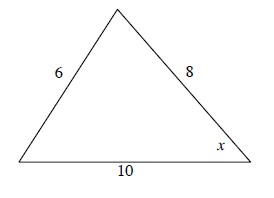2.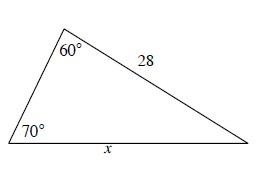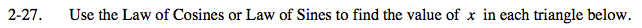Label all of the sides and angles first. Standard labeling is captial letters for angles.
The side opposite the angle is the lower case letter of the angle.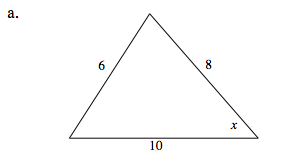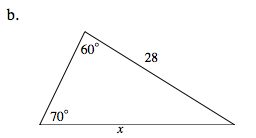$a^2=b^2+c^2-2bc\cos{A}$

$\frac{a}{\sin A}=\frac{b}{\sin B}=\frac{c}{\sin C}$